## Introduction of Paired Mass Distance analysis

pmd package use Paired Mass Distance (PMD) relationship to analysis the GC/LC-MS based non-targeted data. PMD means the distance between two masses or mass to charge ratios. In mass spectrometry, PMD would keep the same value between two masses and two mass to charge ratios(m/z). There are two kinds of PMD involved in this package: PMD from the same compound and PMD from different compounds. In GC/LC-MS or XCMS based non-targeted data analysis, peaks could be separated by chronograph and same compound means ions from similar retention times or ions co-eluted by certain column.

### PMD from the same compound

For MS1 full scan data, we could build retention time(RT) bins to assign peaks into different RT groups by retention time hierarchical clustering analysis. For each RT group, the peaks should come from same compounds or co-elutes. If certain PMD appeared in multiple RT groups, it would be related to the relationship about adducts, neutral loss, isotopologues or common fragments ions.

### PMD from different compounds

The peaks from different retention time groups would like to be different compounds separated by chronograph. The PMD would reflect the relationship about homologous series or chemical reactions.

GlobalStd algorithm use the PMD within same RT group to find independent peaks among certain data set. Then, structure/reaction directed analysis use PMD from different RT groups to screen important compounds or reactions.

## Data format

The input data should be a list object with at least two elements from a peaks list:

• mass to charge ratio with name of mz, high resolution mass spectrometry is required
• retention time with name of rt

However, I suggested to add intensity and group information to the list for validation of PMD analysis.

In this package, a data set from in vivo solid phase micro-extraction(SPME) was attached. This data set contain 9 samples from 3 fish with triplicates samples for each fish. Here is the data structure:

library(pmd)
data("spmeinvivo")
str(spmeinvivo)
#> List of 4
#>  $data : num [1:1459, 1:9] 1095 10439 10154 2797 90211 ... #> ..- attr(*, "dimnames")=List of 2 #> .. ..$ : chr [1:1459] "100.1/170" "100.5/86" "101/85" "103.1/348" ...
#>   .. ..$: chr [1:9] "1405_Fish1_F1" "1405_Fish1_F2" "1405_Fish1_F3" "1405_Fish2_F1" ... #>$ group:'data.frame':   9 obs. of  2 variables:
#>   ..$sample_name : chr [1:9] "1405_Fish1_F1" "1405_Fish1_F2" "1405_Fish1_F3" "1405_Fish2_F1" ... #> ..$ sample_group: chr [1:9] "fish1" "fish1" "fish1" "fish2" ...
#>  $mz : num [1:1459] 100 101 101 103 104 ... #>$ rt   : num [1:1459] 170.2 86.3 84.9 348.1 48.8 ...

You could build this list or mzrt object from the xcms objects via enviGCMS package. When you have a xcmsSet object or XCMSnExp object named xset, you could use enviGCMS::getmzrt(xset) to get such list. Of course you could build such list by yourself.

## GlobalStd algorithm

GlobalStd algorithm try to find independent peaks among certain peaks list. The first step is retention time hierarchical clustering analysis. The second step is to find the relationship among adducts, neutral loss, isotopologues and common fragments ions. The third step is to screen the independent peaks.

Here is a workflow for this algorithm:

knitr::include_graphics('https://yufree.github.io/presentation/figure/GlobalStd.png')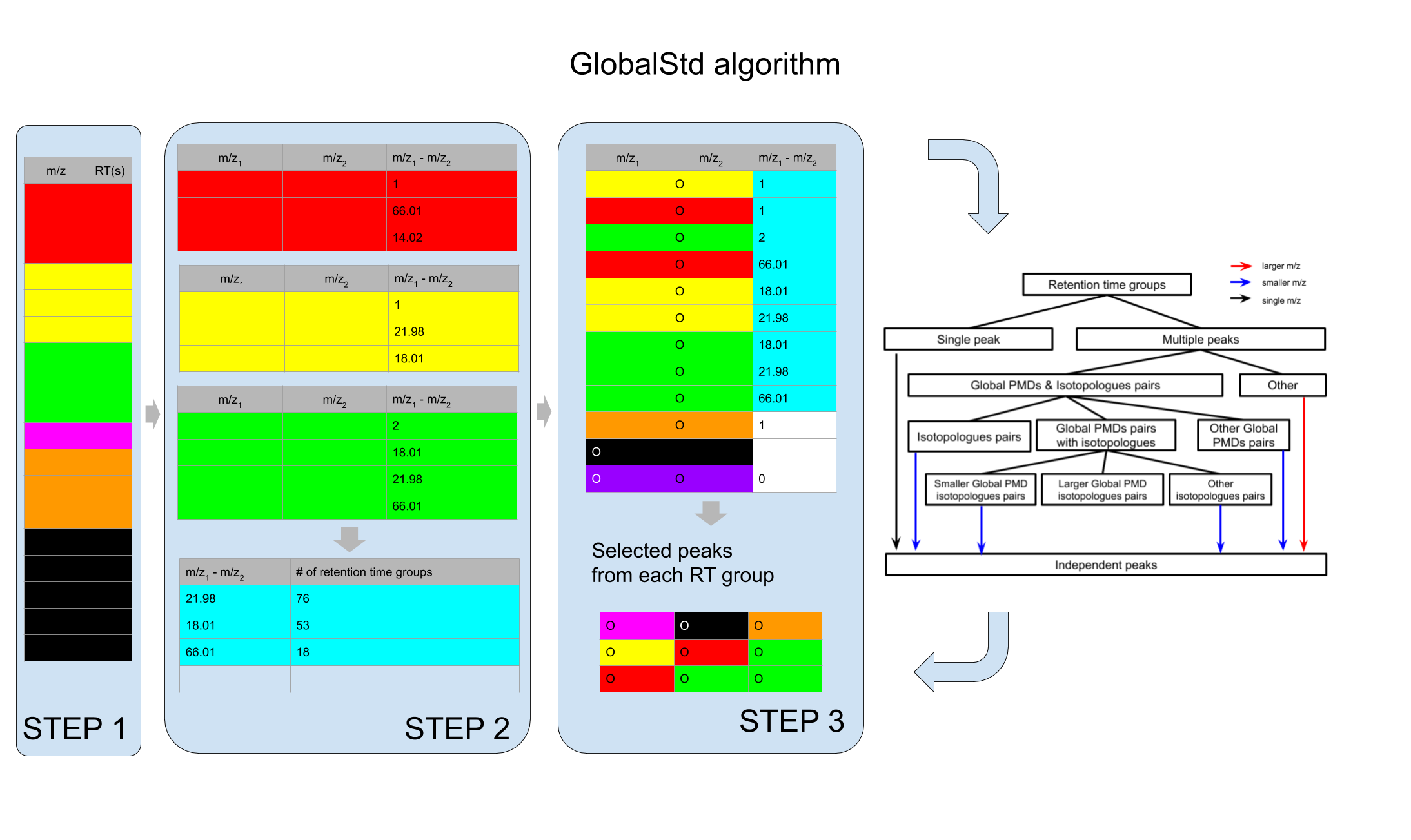### STEP1: Retention time hierarchical clustering

pmd <- getpaired(spmeinvivo)
#> 75 retention time cluster found.
#> 369 paired masses found
#> 5 unique within RT clusters high frequency PMD(s) used for further investigation.
#> The unique within RT clusters high frequency PMD(s) is(are)  28.03 21.98 44.03 17.03 18.01.
#> 719 isotopologue(s) related paired mass found.
#> 492 multi-charger(s) related paired mass found.
plotrtg(pmd)This plot would show the distribution of RT groups. The rtcutoff in function getpaired could be used to set the cutoff of the distances in retention time hierarchical clustering analysis. Retention time cluster cutoff should fit the peak picking algorithm. For HPLC, 10 is suggested and 5 could be used for UPLC.

Global PMD’s retention time group numbers should be around 20 percent of the retention time cluster numbers. For example, if you find 100 retention time clusters, I suggested you use 20 as the cutoff of empirical global PMD’s retention time group numbers. If you don’t specifically assign a value to ng, the algorithm will select such recommendation by default setting.

Take care of the retention time cluster with lots of peaks. In this case, such cluster could be co-eluted compounds on certain column. It would be wise to trim the retention time window for high quality peaks. Another important hint is that pre-filter your peak list by black samples or other quality control samples. Otherwise the running time would be long and lots of pmd relationship would be just from noise.

### STEP2: Relationship among adducts, neutral loss, isotopologues and common fragments ions

The ng in function getpaired could be used to set cutoff of global PMD’s retention time group numbers. If ng is 10, at least 10 of the retention time groups should contain the shown PMD relationship. You could use plotpaired to show the distribution.

plotpaired(pmd)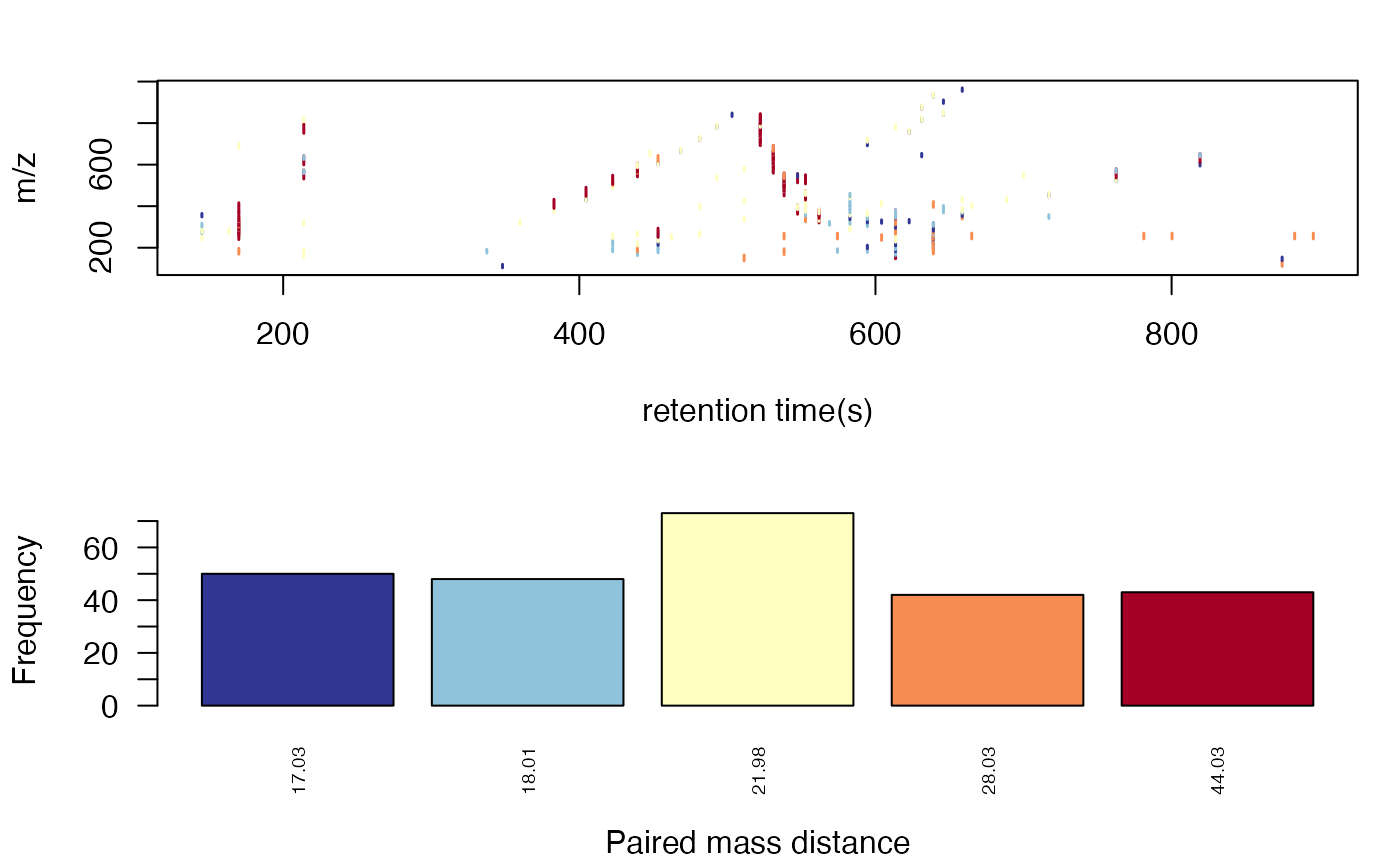You could also show the distribution of PMD relationship by index:

# show the unique PMD found by getpaired function
for(i in 1:length(unique(pmd$paired$diff2))){
diff <- unique(pmd$paired$diff2)[i]
index <- pmd$paired$diff2 == diff
plotpaired(pmd,index)
}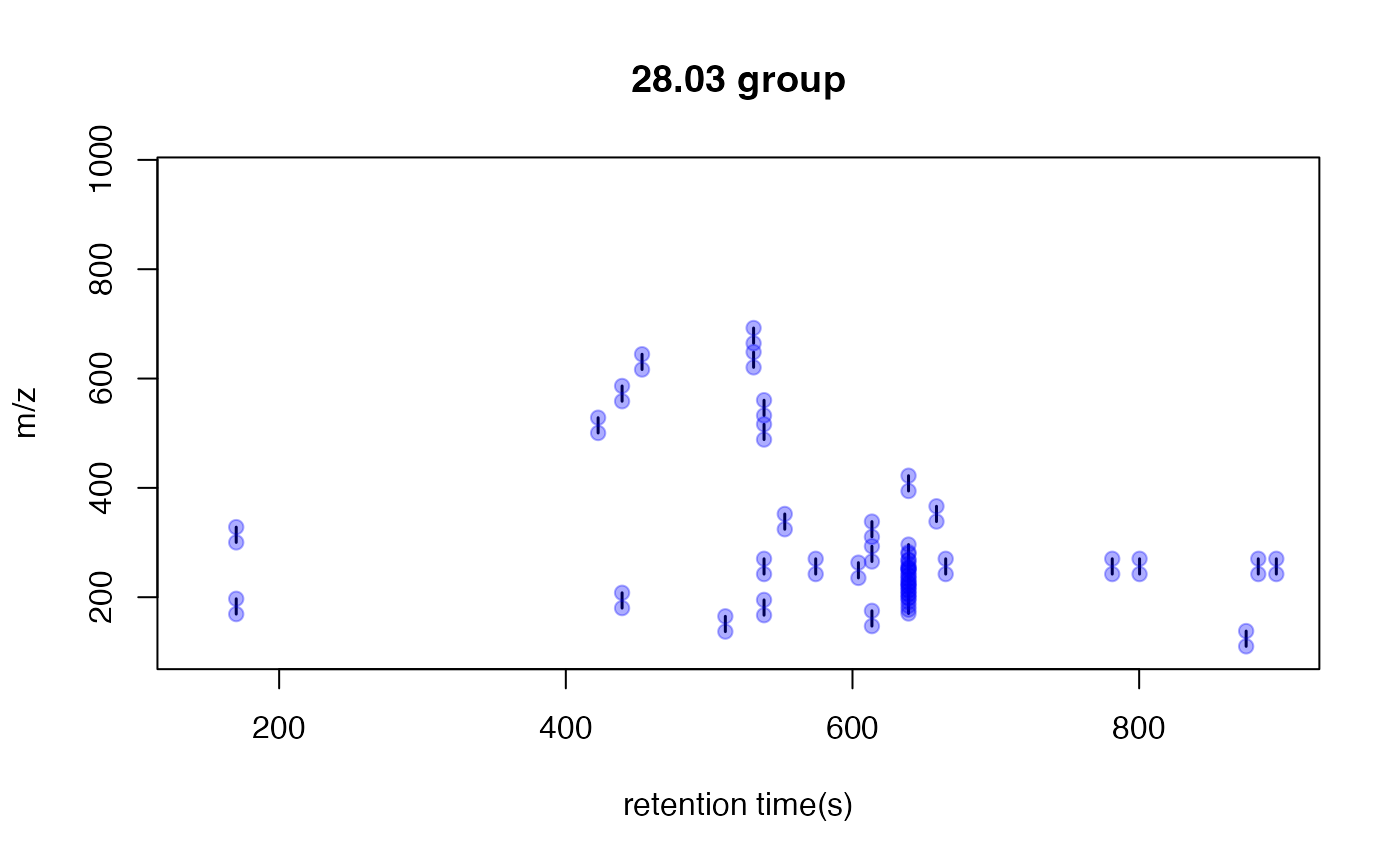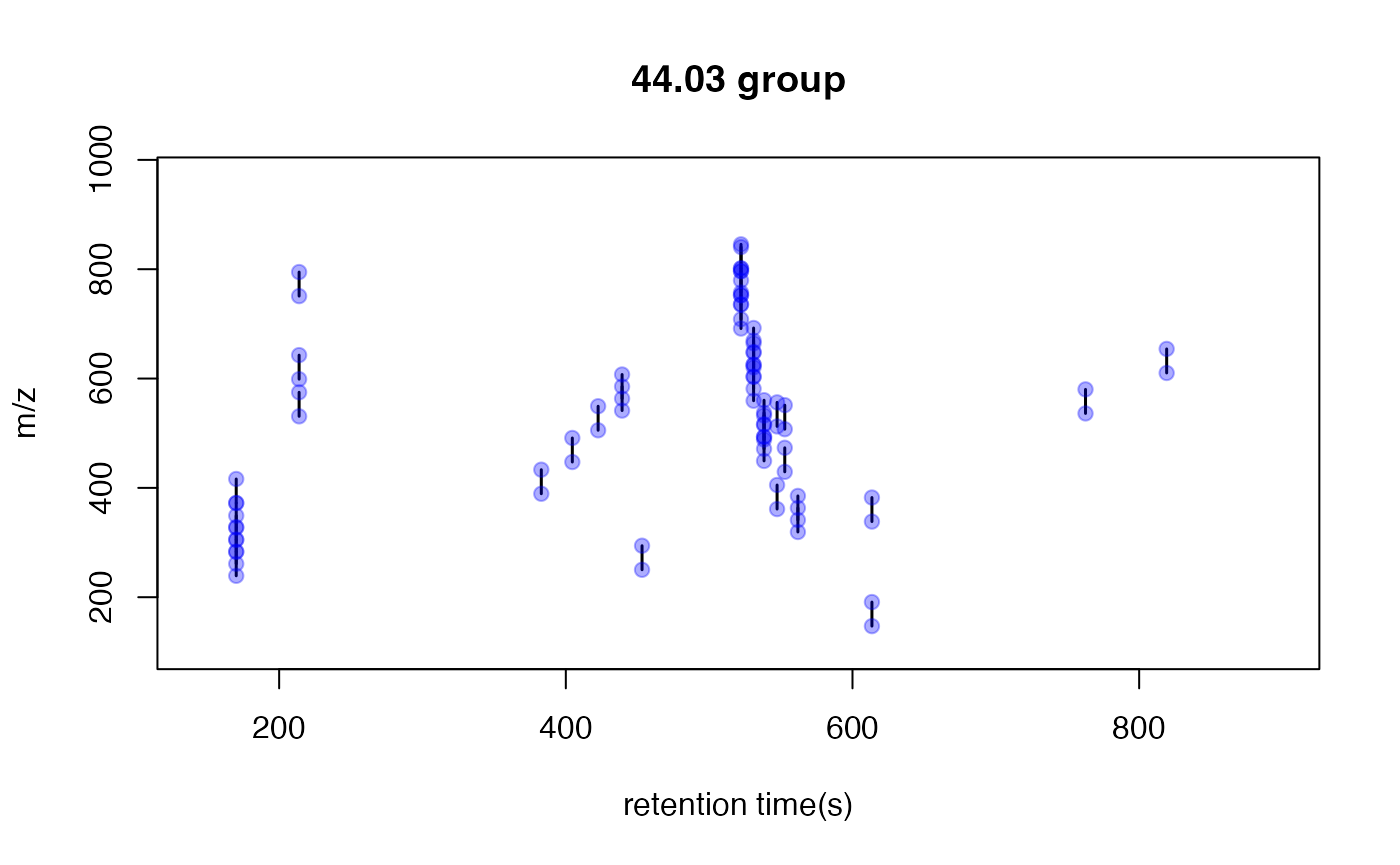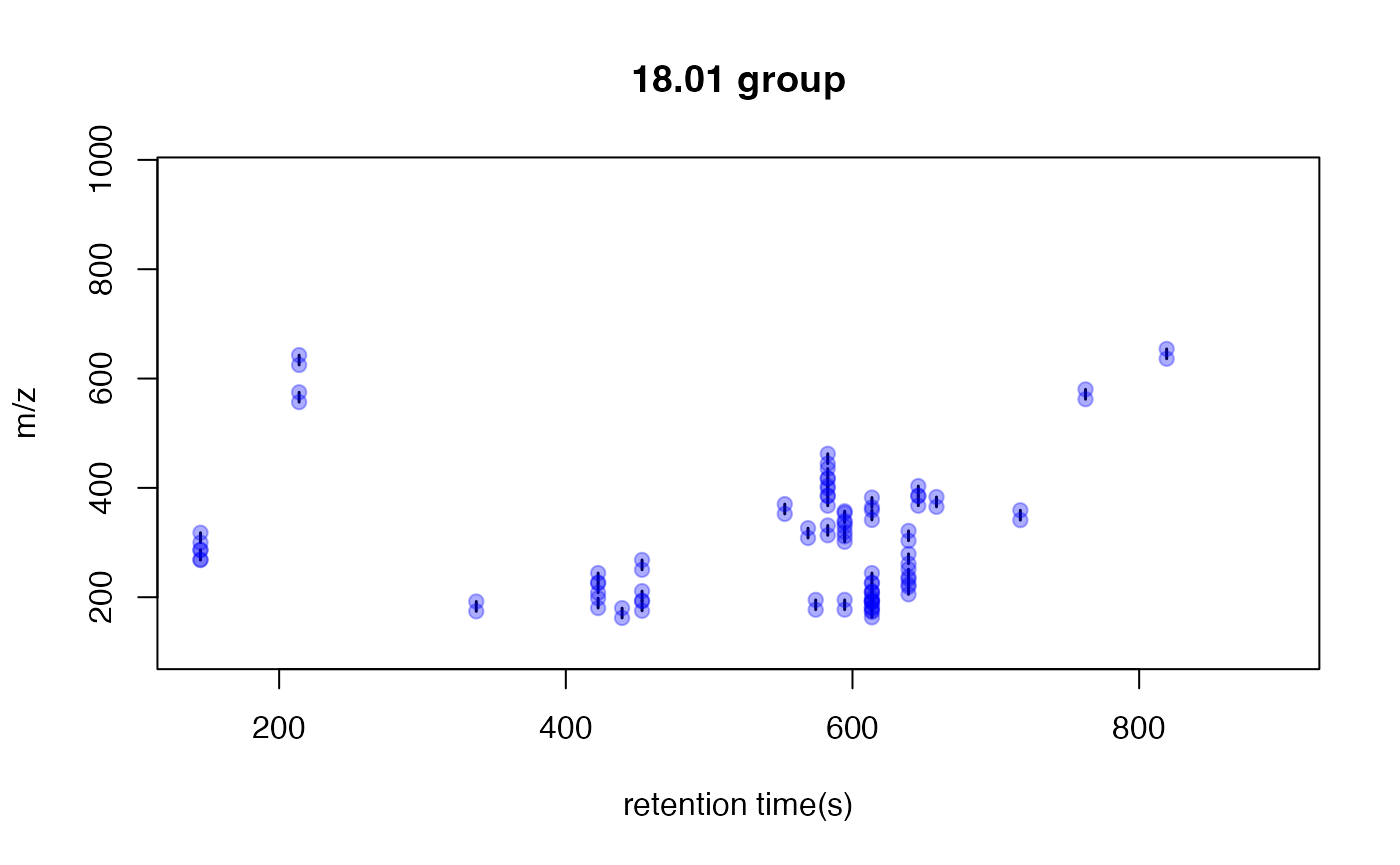This is an easy way to find potential adducts of the data by high frequency PMD from the same compound. For example, 21.98 Da could be the mass distances between $$[M+H]^+$$ and $$[M+Na]^+$$. In this case, user could find the potential adducts or neutral loss even when they have no preferred adducts list. If one adduct exist in certain analytical system, the high frequency PMD will reveal such relationship. The high frequency PMD list could also be used to check the fragmental pattern of in-source reactions as long as such patterns are popular among all collected ions.

### STEP3: Screen the independent peaks

You could use getstd function to get the independent peaks. Independent peaks mean the peaks list removing the redundant peaks such as adducts, neutral loss, isotopologues and comment fragments ions found by PMD analysis in STEP2. Ideally, those peaks could be molecular ions while they might still contain redundant peaks.

std <- getstd(pmd)
#> 8 retention group(s) have single peaks. 14 23 32 33 54 55 56 75
#> 11 group(s) with multiple peaks while no isotope/paired relationship 4 5 7 8 11 41 42 49 68 72 73
#> 9 group(s) with multiple peaks with isotope without paired relationship 2 9 22 26 52 62 64 66 70
#> 4 group(s) with paired relationship without isotope 1 10 15 18
#> 43 group(s) with paired relationship and isotope 3 6 12 13 16 17 19 20 21 24 25 27 28 29 30 31 34 35 36 37 38 39 40 43 44 45 46 47 48 50 51 53 57 58 59 60 61 63 65 67 69 71 74
#> 291 std mass found.

Here you could plot the peaks by plotstd function to show the distribution of independent peaks:

plotstd(std)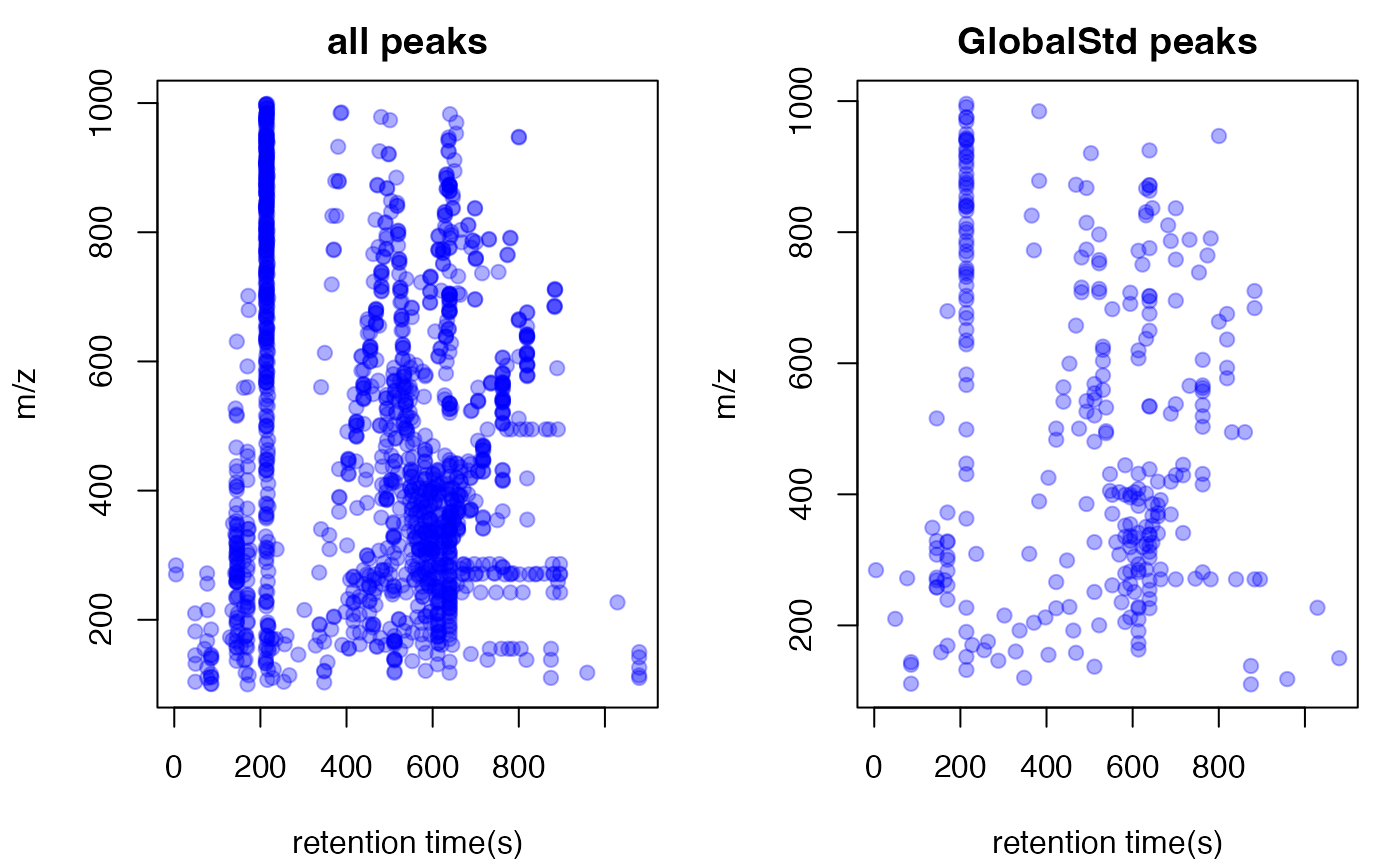You could also plot the peaks distribution by assign a retention time group via plotstdrt:

par(mfrow = c(2,3))
plotstdrt(std,rtcluster = 23,main = 'Retention time group 23')
plotstdrt(std,rtcluster = 9,main = 'Retention time group 9')
plotstdrt(std,rtcluster = 18,main = 'Retention time group 18')
plotstdrt(std,rtcluster = 67,main = 'Retention time group 67')
plotstdrt(std,rtcluster = 49,main = 'Retention time group 49')
plotstdrt(std,rtcluster = 6,main = 'Retention time group 6')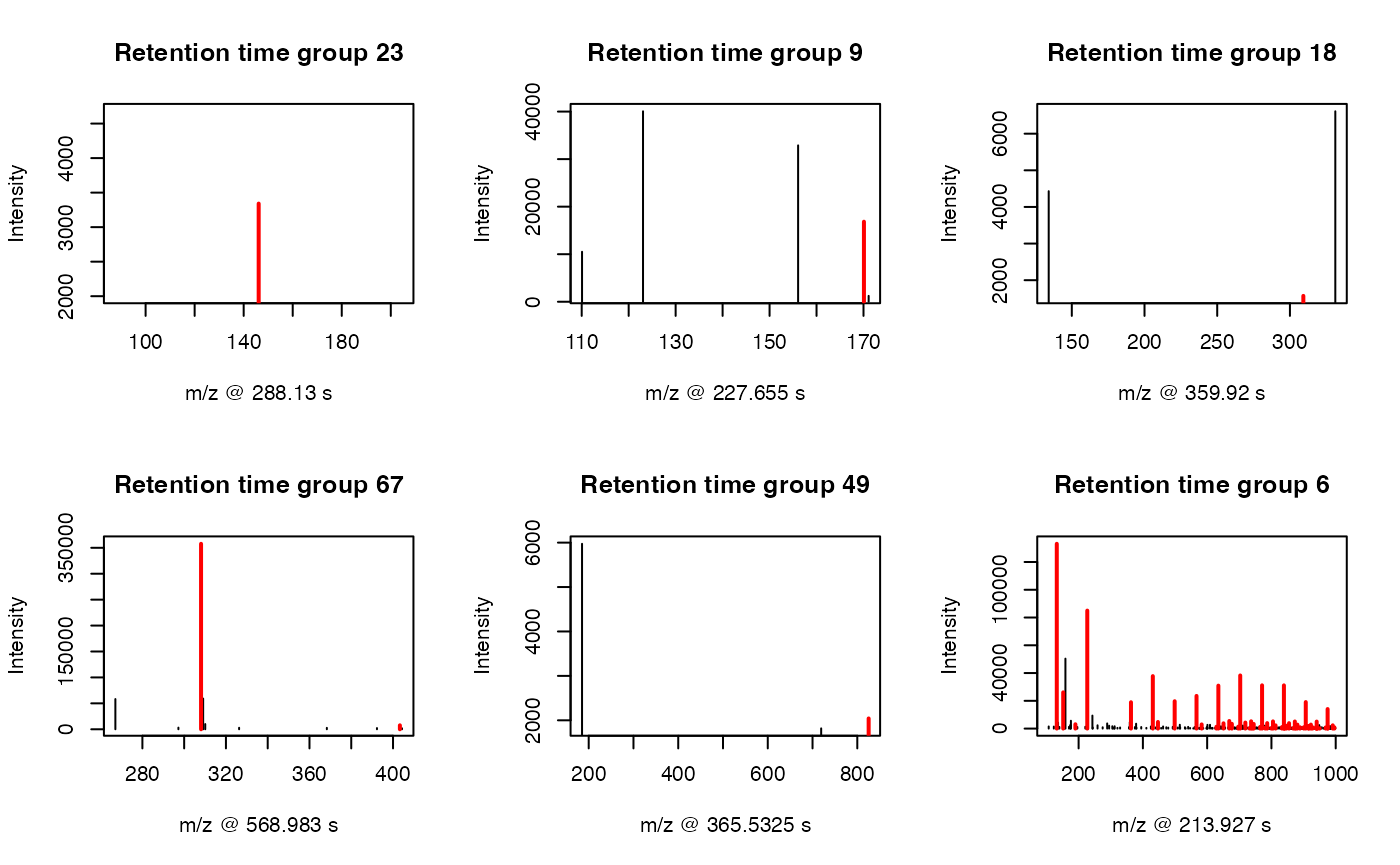### Extra filter with correlation coefficient cutoff

Original GlobalStd algorithm only use mass to charge ratio and retention time of peaks to select independent peaks. However, if intensity data across samples are available, correlation coefficient of paired ions could be used to further filter the random noise in high frequency PMDs. You could set up cutoff of Pearson Correlation Coefficient between peaks to refine the peaks selected by GlobalStd within same retention time groups. In this case, the numbers of selected independent peaks will be further reduced. When you use this parameter, make sure the intensity data are from real samples instead of blank samples, which will affect the calculation of correlation coefficient.

std2 <- getstd(pmd,corcutoff = 0.9)
#> 8 retention group(s) have single peaks. 14 23 32 33 54 55 56 75
#> 23 group(s) with multiple peaks while no isotope/paired relationship 2 4 5 7 8 10 11 15 18 26 35 39 41 42 49 50 59 62 68 69 70 72 73
#> 14 group(s) with multiple peaks with isotope without paired relationship 9 12 22 24 27 28 34 51 52 57 60 64 66 71
#> 3 group(s) with paired relationship without isotope 1 53 74
#> 27 group(s) with paired relationship and isotope 3 6 13 16 17 19 20 21 25 29 30 31 36 37 38 40 43 44 45 46 47 48 58 61 63 65 67
#> 120 std mass found.

## Validation by principal components analysis(PCA)

You need to check the GlobalStd algorithm’s results by principal components analysis(PCA). If we removed too much peaks containing information, the score plot of reduced data set would show great changes.

library(enviGCMS)
par(mfrow = c(2,2),mar = c(4,4,2,1)+0.1)
plotpca(std$data,lv = as.numeric(as.factor(std$group$sample_group)),main = "all peaks") plotpca(std$data[std$stdmassindex,],lv = as.numeric(as.factor(std$group$sample_group)),main = paste(sum(std$stdmassindex),"independent peaks"))
plotpca(std2$data[std2$stdmassindex,],lv = as.numeric(as.factor(std$group$sample_group)),main = paste(sum(std2$stdmassindex),"reduced independent peaks"))You might find original GlobalStd algorithm show a similar PCA score plot with original data while GlobalStd algorithm considering intensity data seems change the profile. The major reason is that correlation coefficient option in the algorithm will remove the paired ions without strong correlation. It will be aggressive to remove low intensity peaks, which are vulnerable by baseline noise. However, such options would be helpful if you only concern high quality peaks for following analysis. Otherwise, original GlobalStd will keep the most information for explorer purpose. ## Comparison with other pseudo spectra extraction method GlobalStd algorithm in pmd package could be treated as a method to extract pseudo spectra. You could use getcluster to get peaks groups information for all GlobalStd peaks. This function would consider the merge of GlobalStd peaks when certain peak is involved in multiple clusters. Then you could choose export peaks with the highest intensities or base peaks in each GlobalStd merged peaks groups. Meanwhile, you could also include the correlation coefficient cutoff to further improve the data quality. stdcluster <- getcluster(std) # extract pseudospectra for std peak 71 idx <- unique(stdcluster$cluster$largei[stdcluster$cluster$i==71]) plot(stdcluster$cluster$mz[stdcluster$cluster$largei==idx],stdcluster$cluster$ins[stdcluster$cluster$largei==idx],type = 'h',xlab = 'm/z',ylab = 'intensity',main = 'pseudo spectra for GlobalStd peak 71')# export peaks with the highest intensities in each GlobalStd peaks groups. data <- stdcluster$data[stdcluster$stdmassindex2,] # considering the correlation coefficient cutoff stdcluster2 <- getcluster(std, corcutoff = 0.9) # considering the correlation coefficient cutoff for both psedospectra extraction and GlobalStd algorithm stdcluster3 <- getcluster(std2, corcutoff = 0.9) We supplied getcorcluster to find peaks groups by correlation analysis only. The base peaks of correlation cluster were selected to stand for the compounds. corcluster <- getcorcluster(spmeinvivo) #> 75 retention time cluster found. # extract pseudospectra 1@46 peak <- corcluster$cluster[corcluster$cluster$largei == '1@46',]
plot(peak$ins~peak$mz,type = 'h',xlab = 'm/z',ylab = 'intensity',main = 'pseudo spectra for correlation cluster')Then we could compare the compare reduced result using PCA similarity factor. A good peak selection algorithm could show a high PCA similarity factor compared with original data set while retain the minimized number of peaks.

par(mfrow = c(3,3),mar = c(4,4,2,1)+0.1)
plotpca(std$data[std$stdmassindex,],lv = as.numeric(as.factor(std$group$sample_group)),main = paste(sum(std$stdmassindex),"independent peaks")) plotpca(std$data[stdcluster$stdmassindex2,],lv = as.numeric(as.factor(std$group$sample_group)),main = paste(sum(stdcluster$stdmassindex2),"independent base peaks"))
plotpca(std$data[stdcluster2$stdmassindex2,],lv = as.numeric(as.factor(std$group$sample_group)),main = paste(sum(stdcluster2$stdmassindex2),"independent reduced base peaks")) plotpca(std$data[corcluster$stdmassindex,],lv = as.numeric(as.factor(std$group$sample_group)),main = paste(sum(corcluster$stdmassindex),"peaks without correlationship"))
plotpca(std$data[corcluster$stdmassindex2,],lv = as.numeric(as.factor(std$group$sample_group)),main = paste(sum(corcluster$stdmassindex2),"base peaks without correlationship")) plotpca(std$data,lv = as.numeric(as.factor(std$group$sample_group)),main = paste(nrow(std$data),"all peaks")) plotpca(std$data[stdcluster3$stdmassindex2,],lv = as.numeric(as.factor(std$group$sample_group)),main = paste(sum(stdcluster3$stdmassindex2),"reduced independent base peaks"))
pcasf(std$data, std$data[std$stdmassindex,]) #> pcasf #> 0.9993497 pcasf(std$data, std$data[stdcluster$stdmassindex2,])
#>     pcasf
#> 0.9993578
pcasf(std$data, std$data[stdcluster2$stdmassindex2,]) #> pcasf #> 0.999346 pcasf(std$data, std$data[corcluster$stdmassindex,])
#>     pcasf
#> 0.9471586
pcasf(std$data, std$data[corcluster$stdmassindex2,]) #> pcasf #> 0.9497193 pcasf(std$data, std$data[stdcluster3$stdmassindex2,])
#>    pcasf
#> 0.713527In this case, five peaks selection algorithms are fine to stand for the original peaks with PCA similarity score larger than 0.9. However, the independent base peaks retain the most information with relative low numbers of peaks.

## Structure/Reaction directed analysis

getsda function could be used to perform Structure/reaction directed analysis. The cutoff of frequency is automate found by PMD network analysis with the largest mean distance of all nodes.

sda <- getsda(std)
#> PMD frequency cutoff is 6 by PMD network analysis with largest network average distance 6.67 .
#> 53 groups were found as high frequency PMD group.
#> 0 was found as high frequency PMD.
#> 1.98 was found as high frequency PMD.
#> 2.01 was found as high frequency PMD.
#> 2.02 was found as high frequency PMD.
#> 6.97 was found as high frequency PMD.
#> 11.96 was found as high frequency PMD.
#> 12 was found as high frequency PMD.
#> 13.98 was found as high frequency PMD.
#> 14.02 was found as high frequency PMD.
#> 14.05 was found as high frequency PMD.
#> 15.99 was found as high frequency PMD.
#> 16.03 was found as high frequency PMD.
#> 19.04 was found as high frequency PMD.
#> 28.03 was found as high frequency PMD.
#> 30.05 was found as high frequency PMD.
#> 31.99 was found as high frequency PMD.
#> 33.02 was found as high frequency PMD.
#> 37.02 was found as high frequency PMD.
#> 42.05 was found as high frequency PMD.
#> 48.04 was found as high frequency PMD.
#> 48.98 was found as high frequency PMD.
#> 49.02 was found as high frequency PMD.
#> 54.05 was found as high frequency PMD.
#> 56.06 was found as high frequency PMD.
#> 56.1 was found as high frequency PMD.
#> 58.04 was found as high frequency PMD.
#> 58.08 was found as high frequency PMD.
#> 58.11 was found as high frequency PMD.
#> 63.96 was found as high frequency PMD.
#> 66.05 was found as high frequency PMD.
#> 68.06 was found as high frequency PMD.
#> 70.04 was found as high frequency PMD.
#> 70.08 was found as high frequency PMD.
#> 74.02 was found as high frequency PMD.
#> 80.03 was found as high frequency PMD.
#> 82.08 was found as high frequency PMD.
#> 88.05 was found as high frequency PMD.
#> 91.1 was found as high frequency PMD.
#> 93.12 was found as high frequency PMD.
#> 94.1 was found as high frequency PMD.
#> 96.09 was found as high frequency PMD.
#> 101.05 was found as high frequency PMD.
#> 108.13 was found as high frequency PMD.
#> 110.11 was found as high frequency PMD.
#> 112.16 was found as high frequency PMD.
#> 116.08 was found as high frequency PMD.
#> 122.15 was found as high frequency PMD.
#> 124.16 was found as high frequency PMD.
#> 126.14 was found as high frequency PMD.
#> 144.18 was found as high frequency PMD.
#> 148.04 was found as high frequency PMD.
#> 150.2 was found as high frequency PMD.
#> 173.18 was found as high frequency PMD.

Such largest mean distance of all nodes is calculated for top 1 to 100 (if possible) high frequency PMDs. Here is a demo for the network generation process.

library(igraph)
#>
#> Attaching package: 'igraph'
#> The following objects are masked from 'package:stats':
#>
#>     decompose, spectrum
#> The following object is masked from 'package:base':
#>
#>     union
cdf <- sda$sda # get the PMDs and frequency pmds <- as.numeric(names(sort(table(cdf$diff2),decreasing = T)))
freq <- sort(table(cdf$diff2),decreasing = T) # filter the frequency larger than 10 for demo pmds <- pmds[freq>10] cdf <- sda$sda[sda$sda$diff2 %in% pmds,]
g <- igraph::graph_from_data_frame(cdf,directed = F)
l <- igraph::layout_with_fr(g)
for(i in 1:length(pmds)){
g2 <- igraph::delete_edges(g,which(E(g)$diff2%in%pmds[1:i])) plot(g2,edge.width=1,vertex.label="",vertex.size=1,layout=l,main=paste('Top',length(pmds)-i,'high frequency PMDs')) }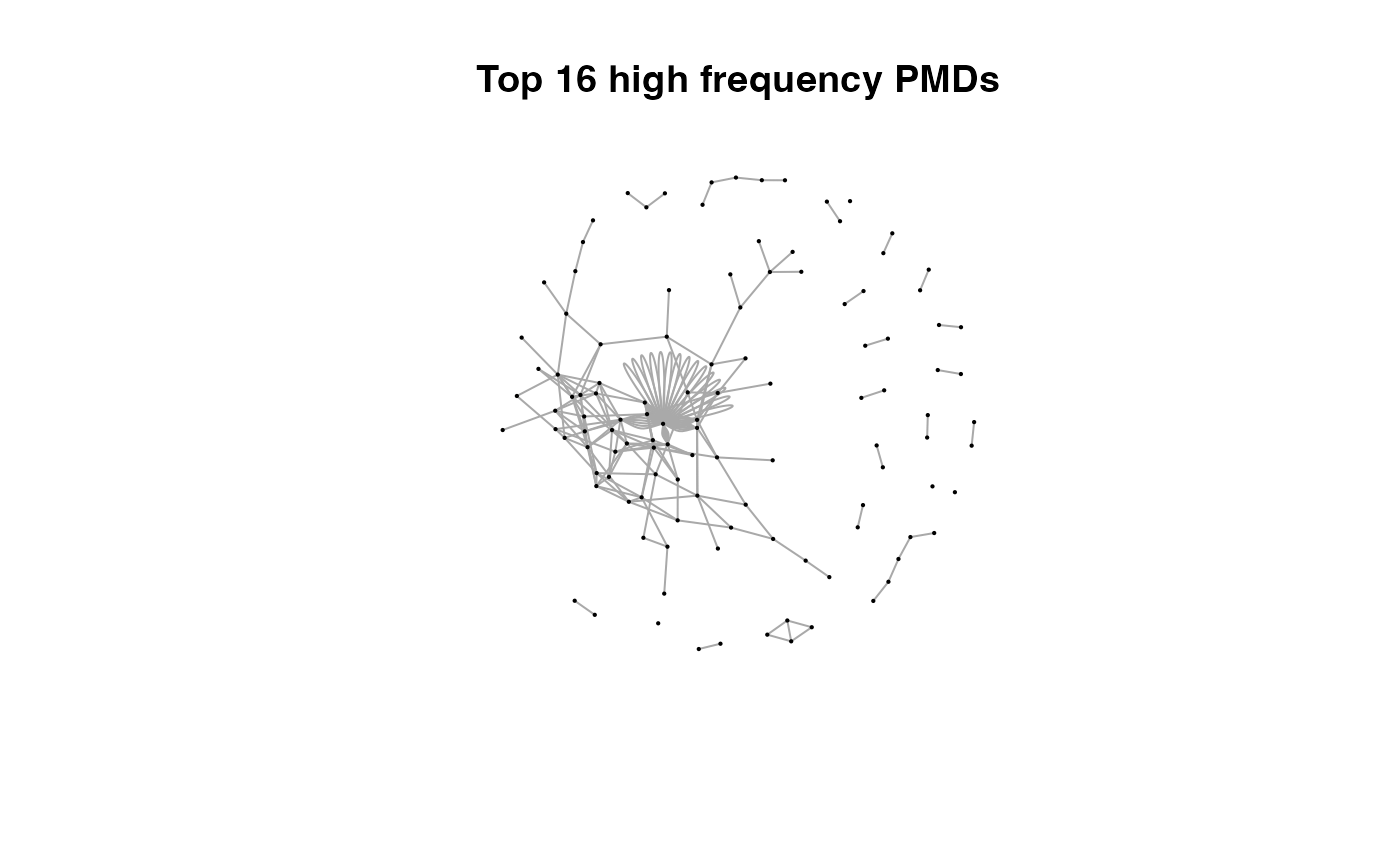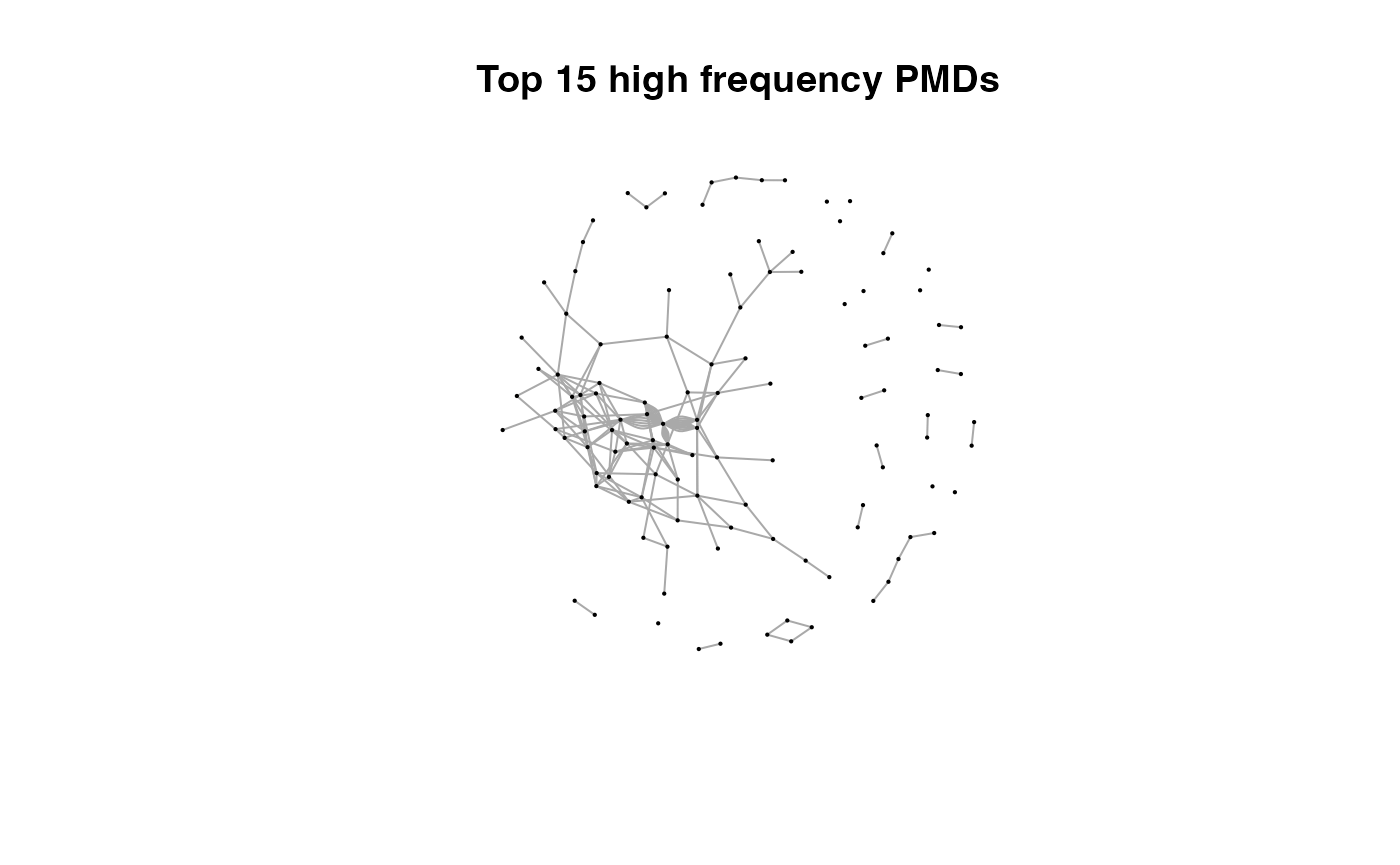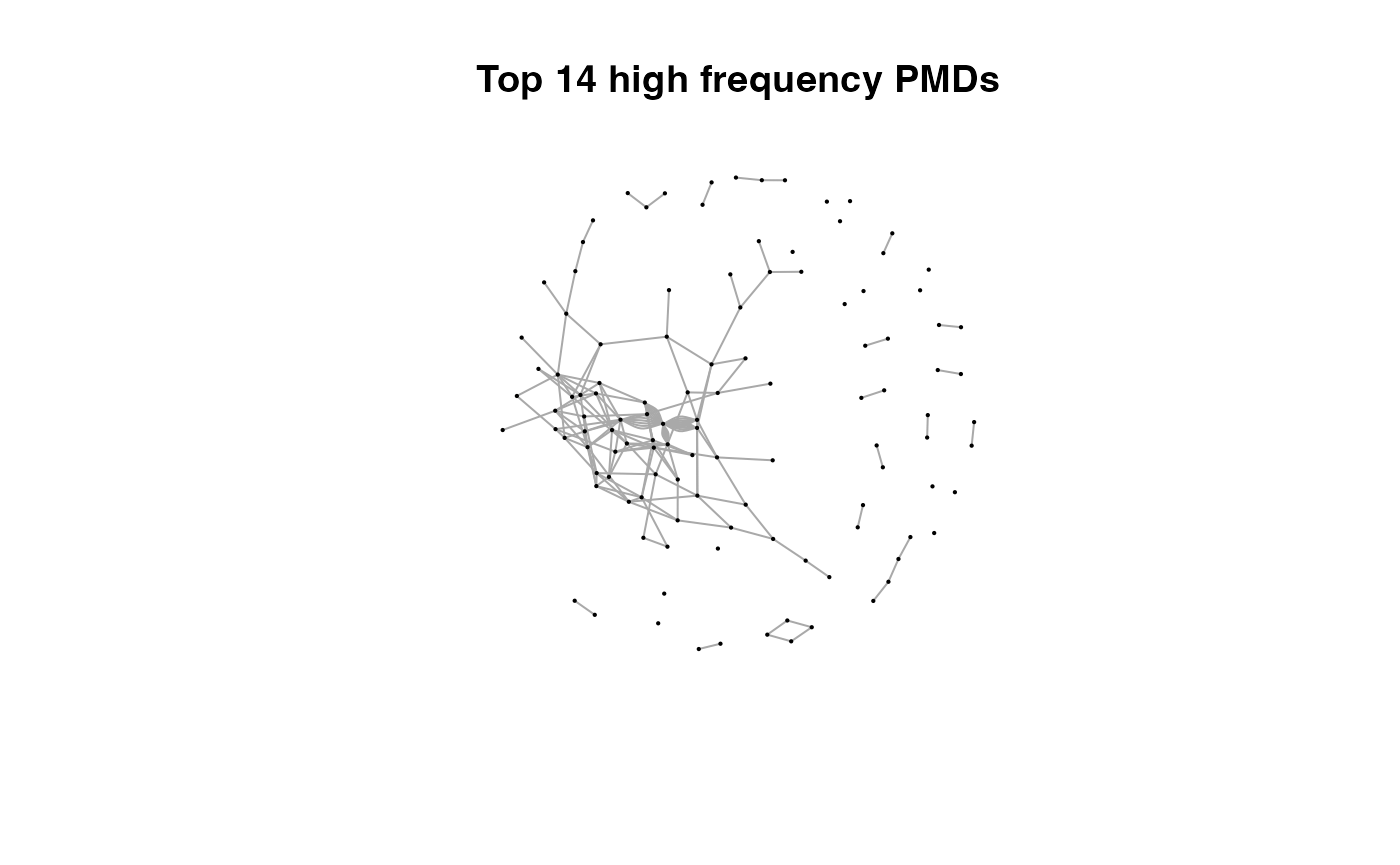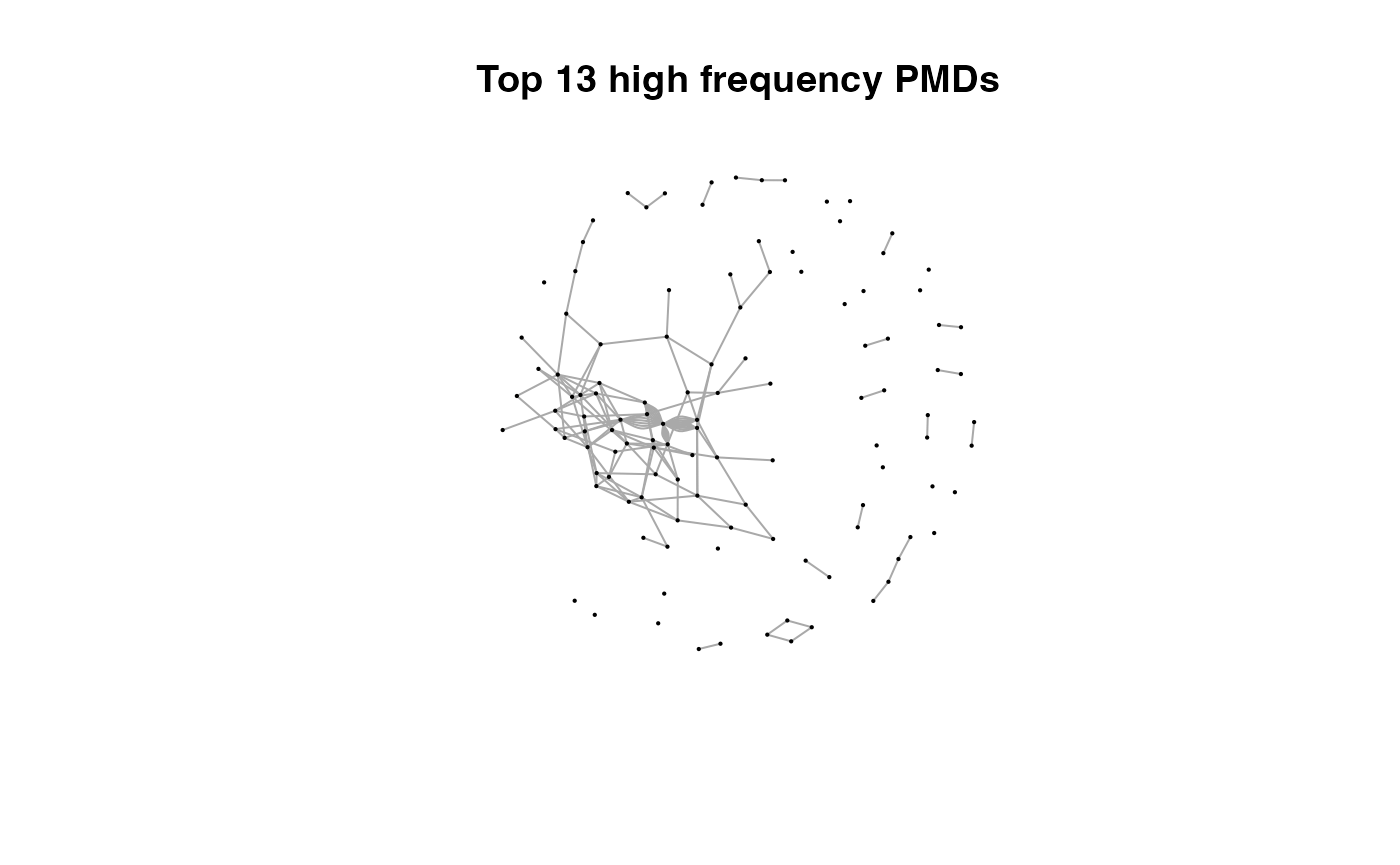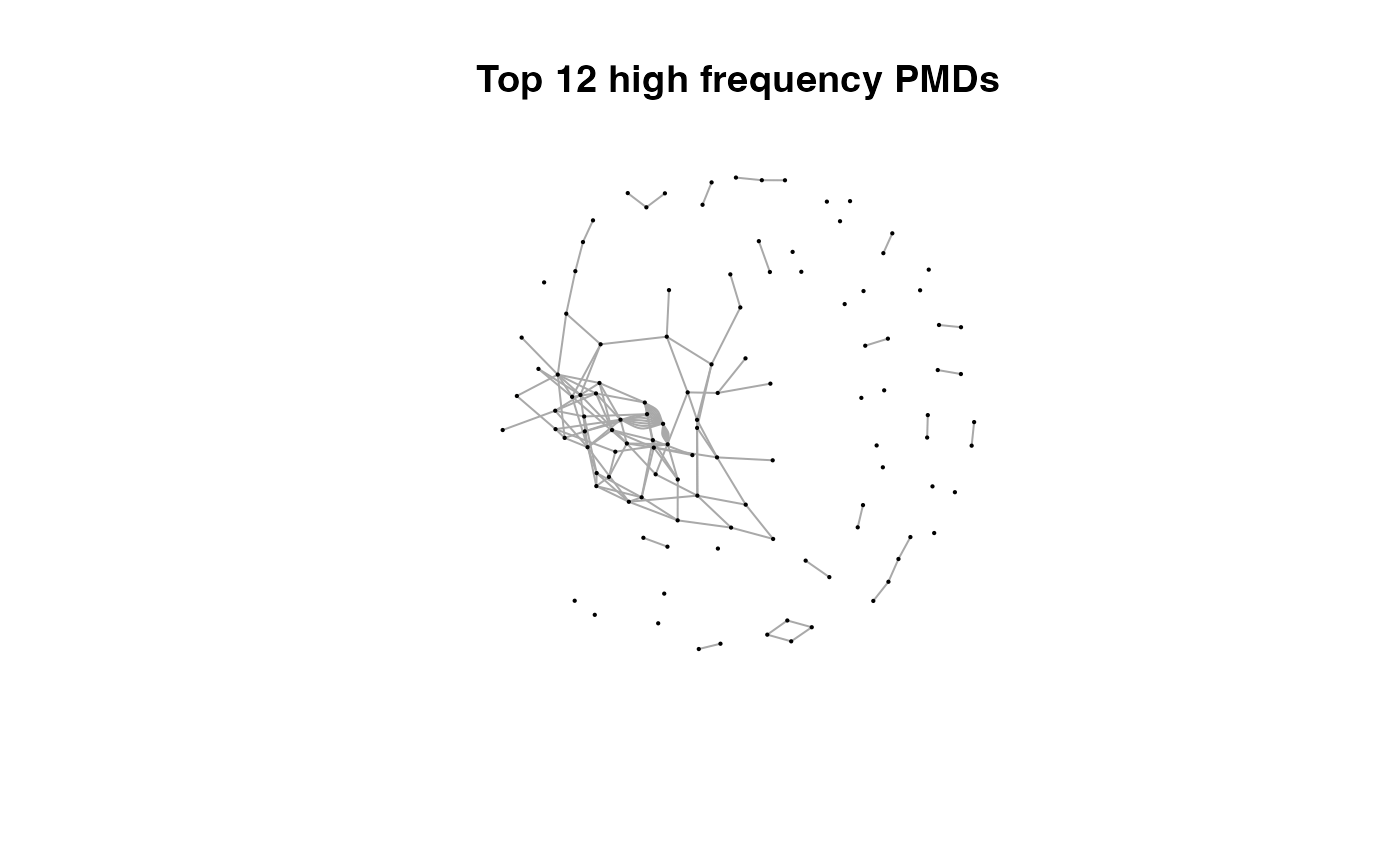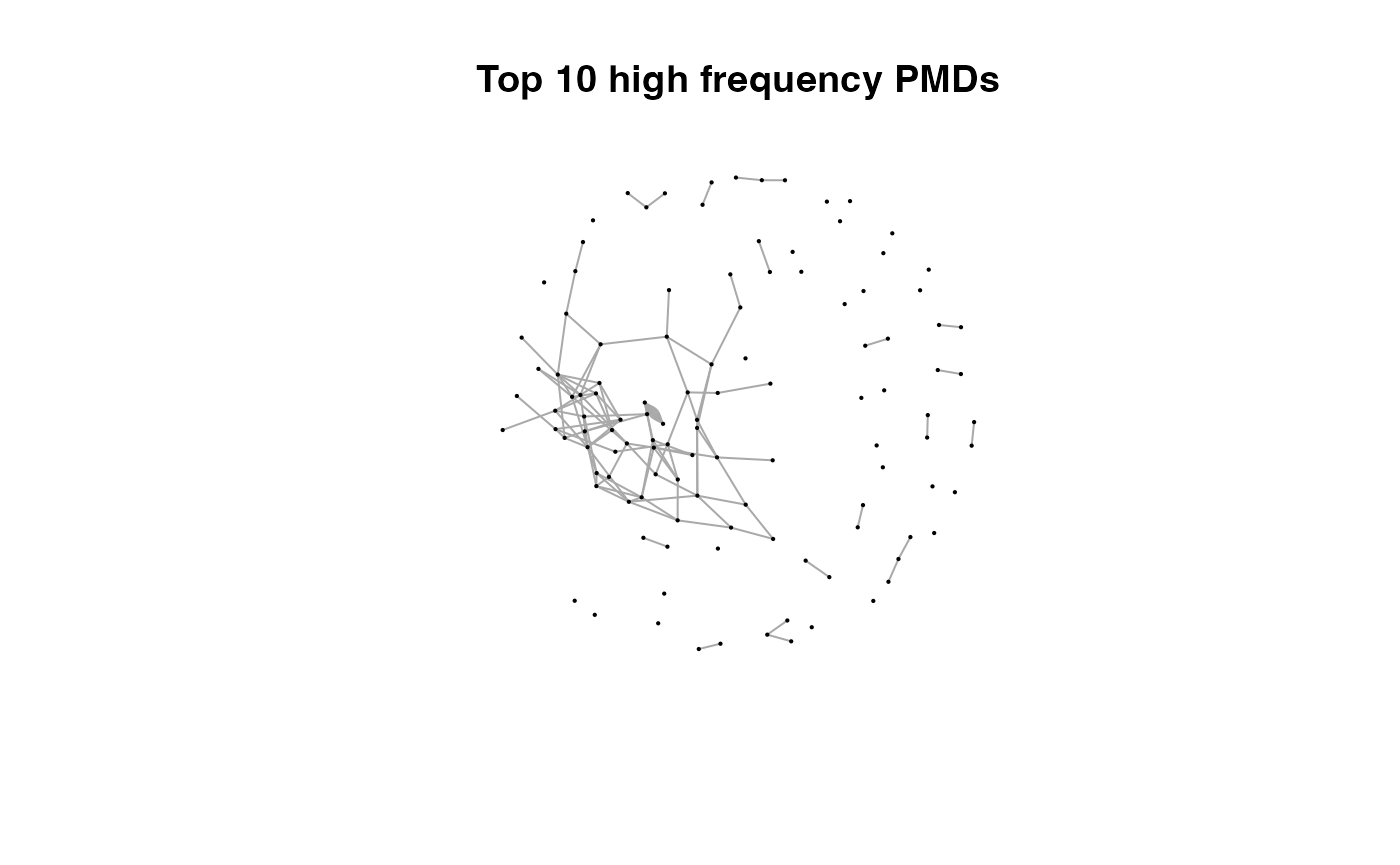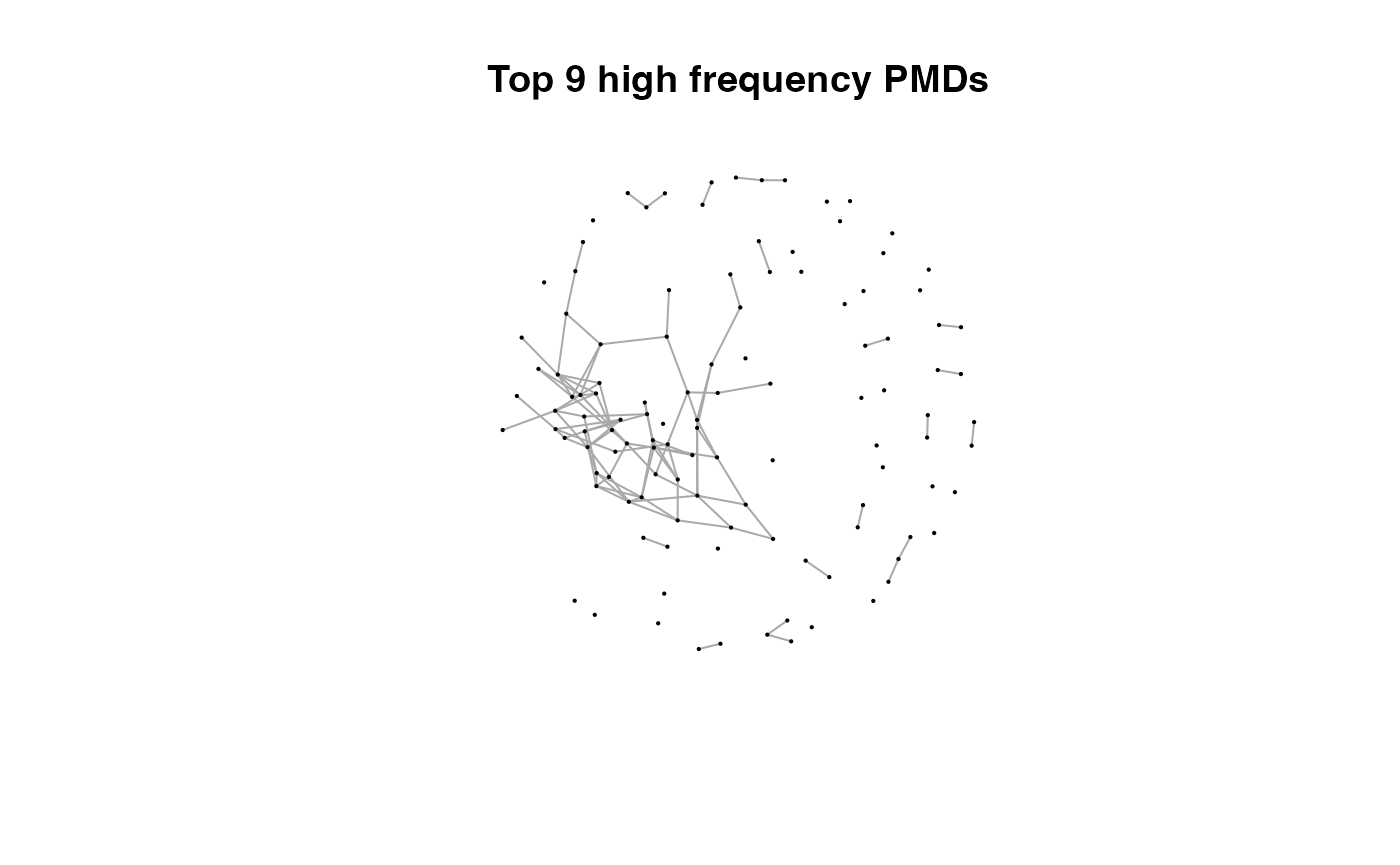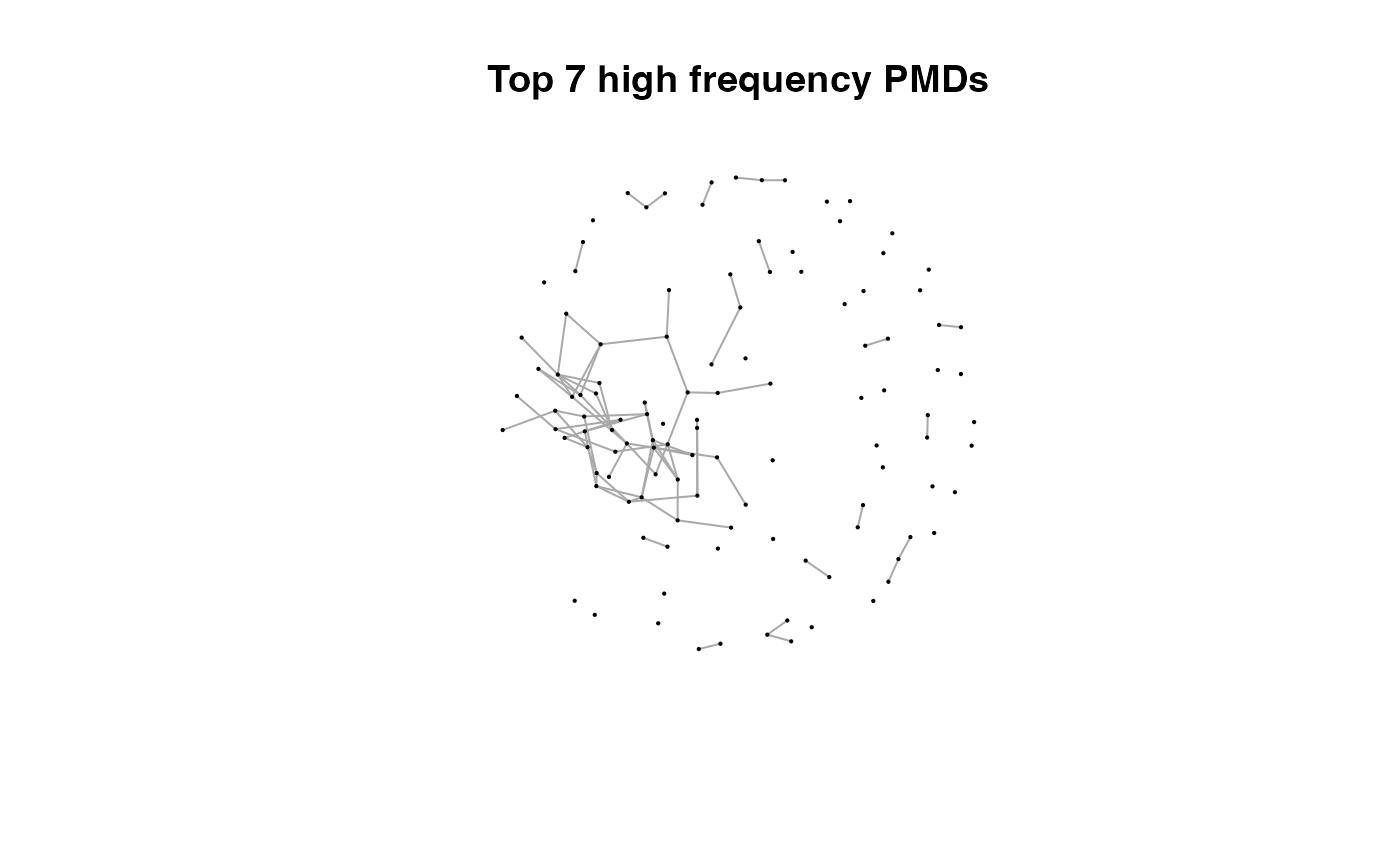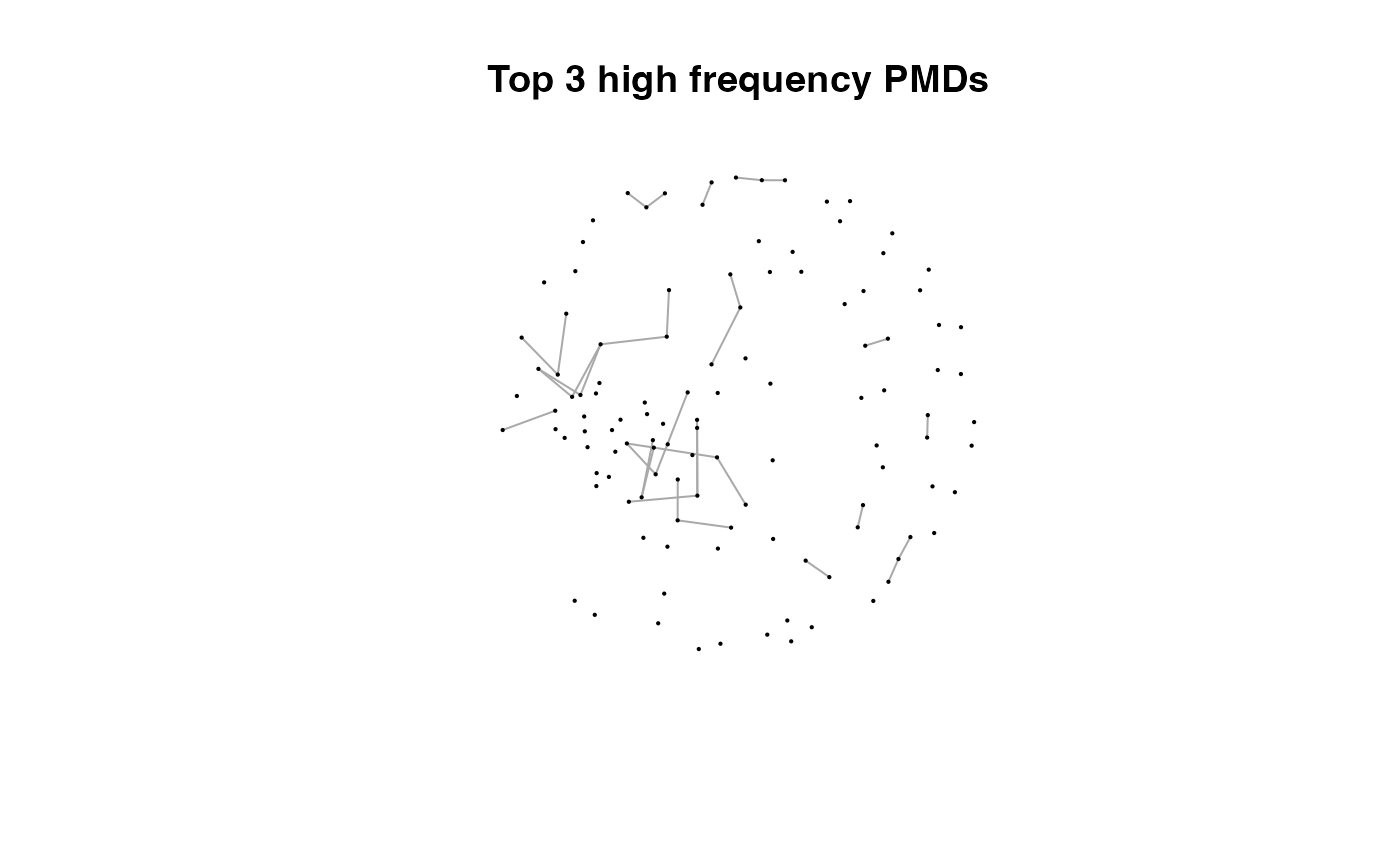Here we could find more and more compounds will be connected with more high frequency PMDs. Meanwhile, the mean distance of all network nodes will increase. However, some PMDs are generated by random combination of ions. In this case, if we included those PMDs for the network, the mean distance of all network nodes will decrease. Here, the largest mean distance means no more information will be found for certain data set and such value is used as the cutoff for high frequency PMDs selection. You could use plotstdsda to show the distribution of the selected paired peaks. plotstdsda(sda)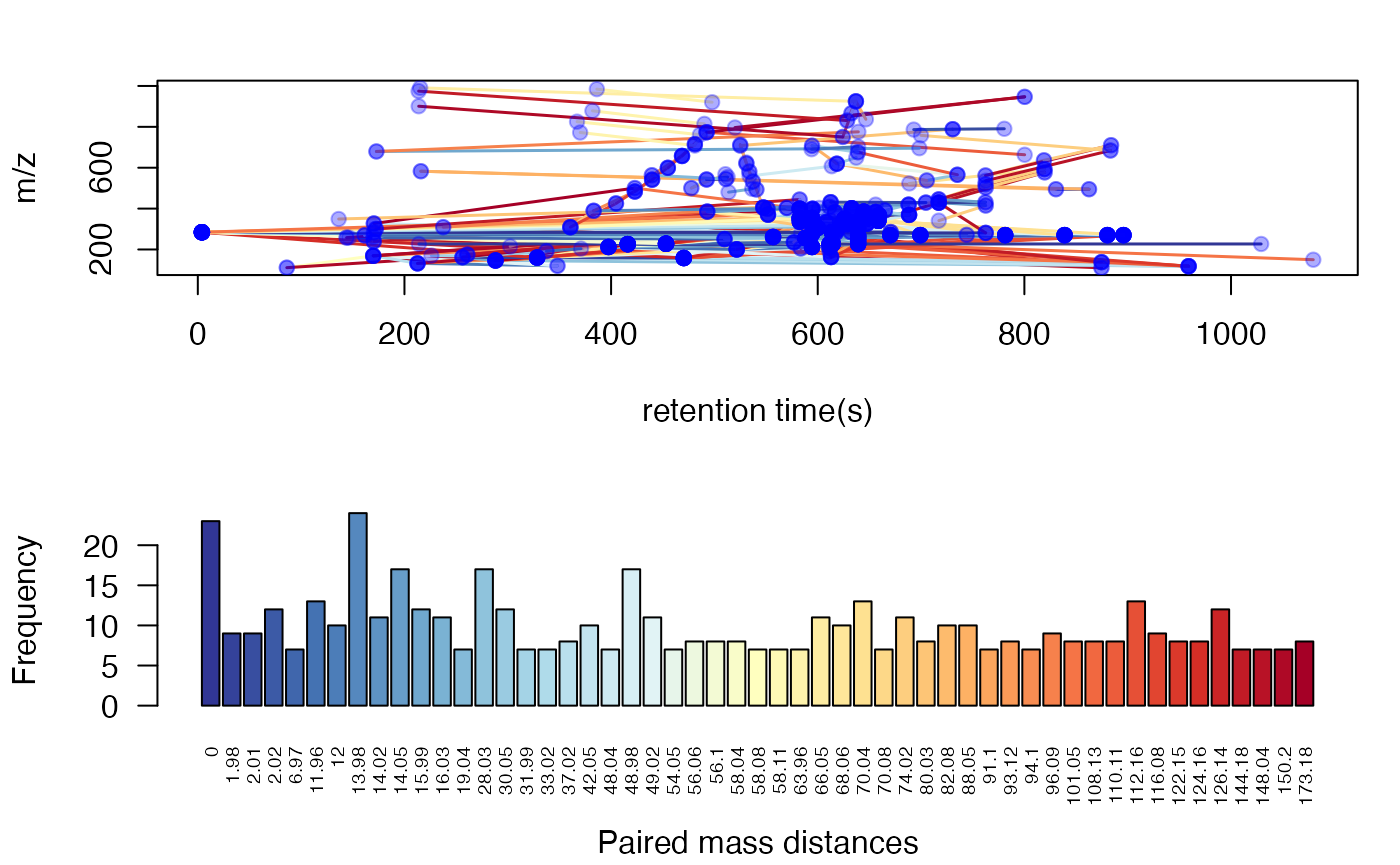You could also use index to show the distribution of certain PMDs. par(mfrow = c(1,3),mar = c(4,4,2,1)+0.1) plotstdsda(sda,sda$sda$diff2 == 2.02) plotstdsda(sda,sda$sda$diff2 == 28.03) plotstdsda(sda,sda$sda$diff2 == 58.04)Structure/reaction directed analysis could be directly performed on all the peaks, which is slow to process: sdaall <- getsda(spmeinvivo) #> PMD frequency cutoff is 104 by PMD network analysis with largest network average distance 14.06 . #> 6 groups were found as high frequency PMD group. #> 0 was found as high frequency PMD. #> 2.02 was found as high frequency PMD. #> 28.03 was found as high frequency PMD. #> 31.01 was found as high frequency PMD. #> 58.04 was found as high frequency PMD. #> 116.08 was found as high frequency PMD. par(mfrow = c(1,3),mar = c(4,4,2,1)+0.1) plotstdsda(sdaall,sdaall$sda$diff2 == 2.02) plotstdsda(sdaall,sdaall$sda$diff2 == 28.03) plotstdsda(sdaall,sdaall$sda$diff2 == 58.04)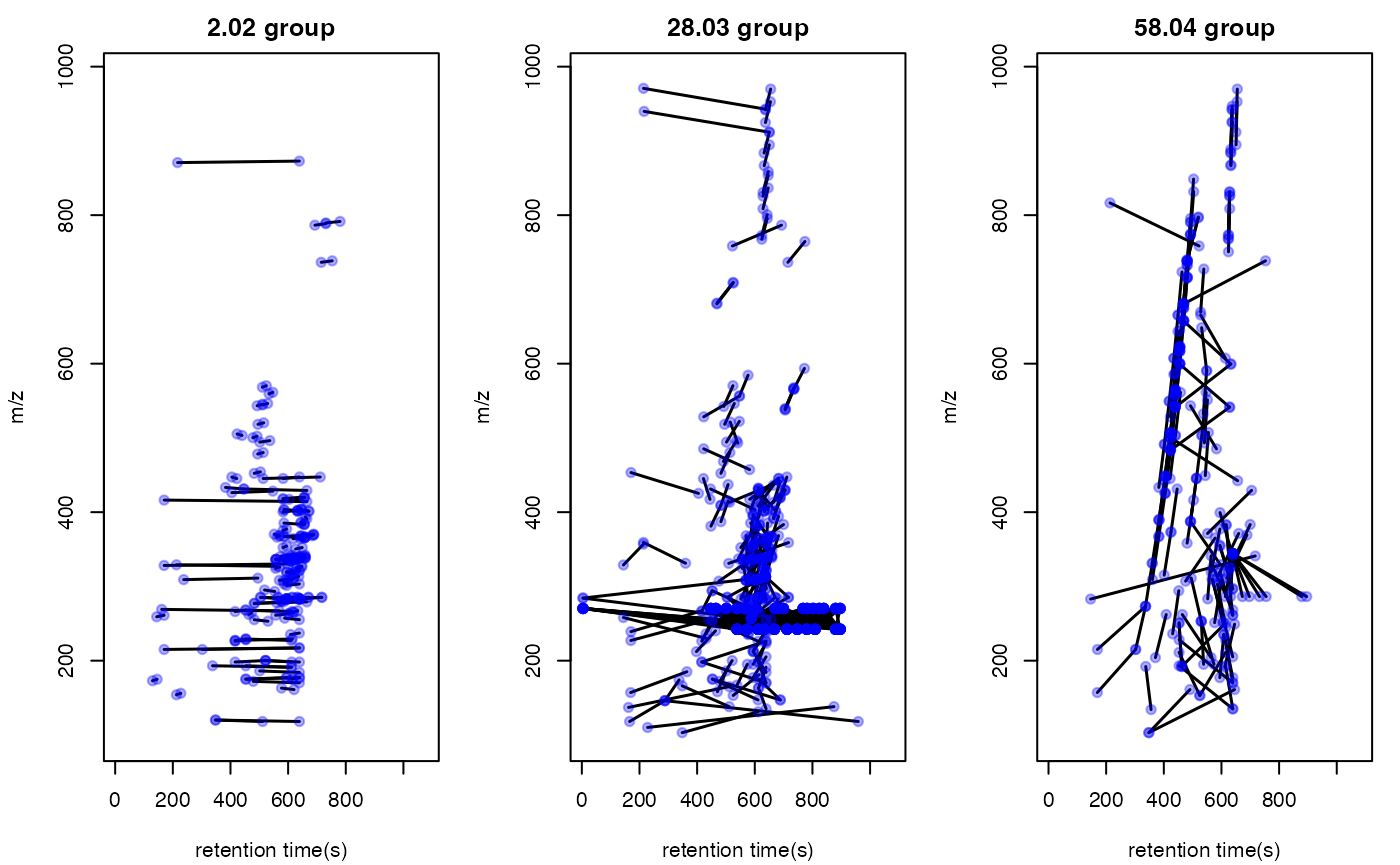### Extra filter with correlation coefficient cutoff Structure/Reaction directed analysis could also use correlation to restrict the paired ions. However, similar to GlobalStd algorithm, such cutoff will remove low intensity data. Researcher should have a clear idea to use this cutoff. sda2 <- getsda(std, corcutoff = 0.9) #> PMD frequency cutoff is 6 by PMD network analysis with largest network average distance 6.67 . #> 41 groups were found as high frequency PMD group. #> 0 was found as high frequency PMD. #> 1.98 was found as high frequency PMD. #> 2.01 was found as high frequency PMD. #> 2.02 was found as high frequency PMD. #> 11.96 was found as high frequency PMD. #> 12 was found as high frequency PMD. #> 13.98 was found as high frequency PMD. #> 14.02 was found as high frequency PMD. #> 14.05 was found as high frequency PMD. #> 15.99 was found as high frequency PMD. #> 16.03 was found as high frequency PMD. #> 19.04 was found as high frequency PMD. #> 28.03 was found as high frequency PMD. #> 30.05 was found as high frequency PMD. #> 31.99 was found as high frequency PMD. #> 33.02 was found as high frequency PMD. #> 42.05 was found as high frequency PMD. #> 48.98 was found as high frequency PMD. #> 49.02 was found as high frequency PMD. #> 54.05 was found as high frequency PMD. #> 56.06 was found as high frequency PMD. #> 58.04 was found as high frequency PMD. #> 58.08 was found as high frequency PMD. #> 63.96 was found as high frequency PMD. #> 66.05 was found as high frequency PMD. #> 68.06 was found as high frequency PMD. #> 70.08 was found as high frequency PMD. #> 74.02 was found as high frequency PMD. #> 80.03 was found as high frequency PMD. #> 82.08 was found as high frequency PMD. #> 88.05 was found as high frequency PMD. #> 93.12 was found as high frequency PMD. #> 94.1 was found as high frequency PMD. #> 96.09 was found as high frequency PMD. #> 108.13 was found as high frequency PMD. #> 110.11 was found as high frequency PMD. #> 112.16 was found as high frequency PMD. #> 116.08 was found as high frequency PMD. #> 122.15 was found as high frequency PMD. #> 124.16 was found as high frequency PMD. #> 126.14 was found as high frequency PMD. plotstdsda(sda2)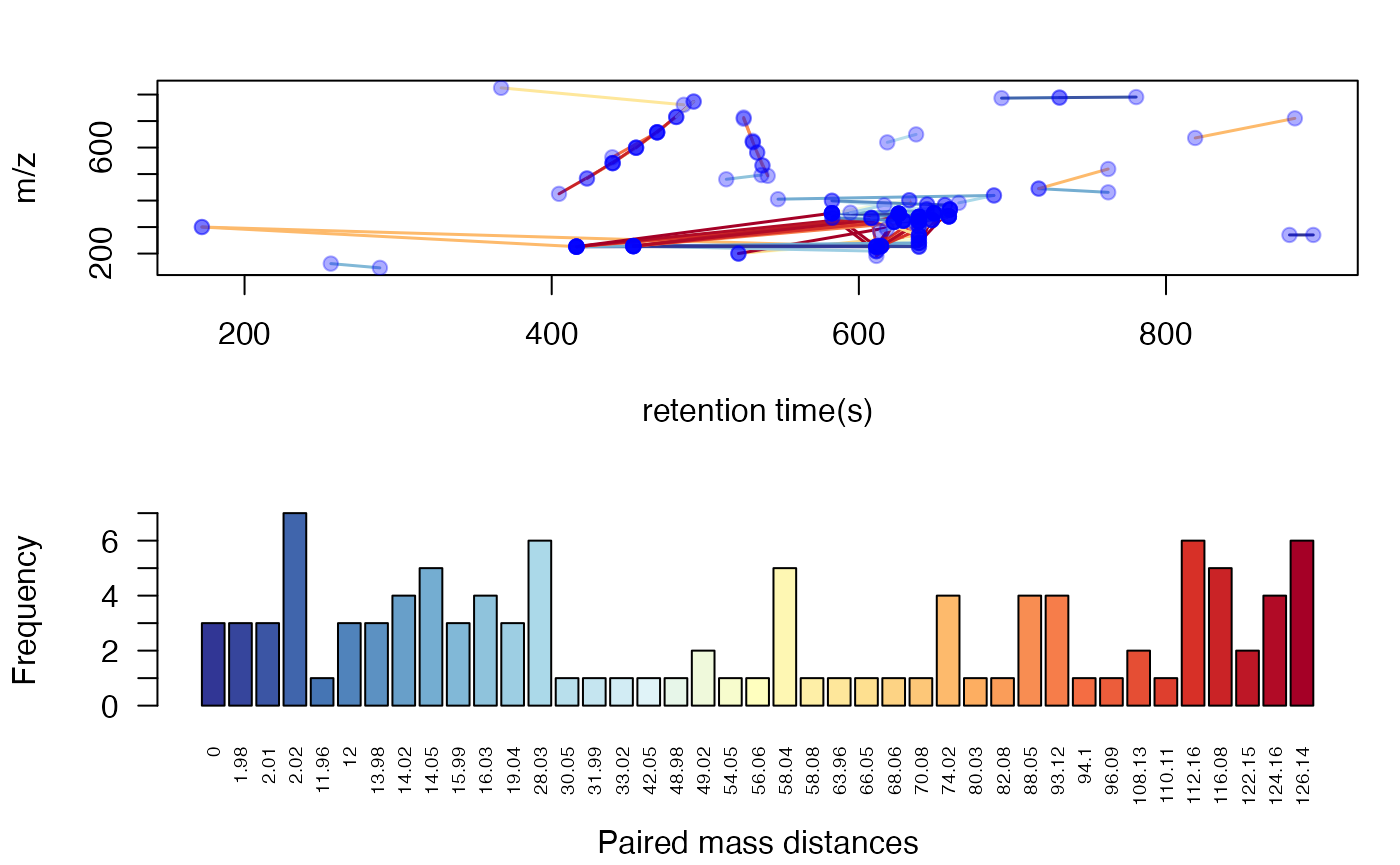### Structure/reaction directed analysis for peaks/compounds only data When you only have data of peaks without retention time or compounds list, structure/reaction directed analysis could also be done by getrda function. sda <- getrda(spmeinvivo$mz[std$stdmassindex]) #> 15209 pmd found. #> 3 pmd used. ## Wrap function for GlobalStd algorithm globalstd function is a wrap function to process GlobalStd algorithm and structure/reaction directed analysis in one line. All the plot function could be directly used on the list objects from globalstd function. If you want to perform structure/reaction directed analysis, set the sda=T in the globalstd function. result <- globalstd(spmeinvivo, sda=FALSE) #> 75 retention time cluster found. #> 369 paired masses found #> 5 unique within RT clusters high frequency PMD(s) used for further investigation. #> The unique within RT clusters high frequency PMD(s) is(are) 28.03 21.98 44.03 17.03 18.01. #> 719 isotopologue(s) related paired mass found. #> 492 multi-charger(s) related paired mass found. #> 8 retention group(s) have single peaks. 14 23 32 33 54 55 56 75 #> 11 group(s) with multiple peaks while no isotope/paired relationship 4 5 7 8 11 41 42 49 68 72 73 #> 9 group(s) with multiple peaks with isotope without paired relationship 2 9 22 26 52 62 64 66 70 #> 4 group(s) with paired relationship without isotope 1 10 15 18 #> 43 group(s) with paired relationship and isotope 3 6 12 13 16 17 19 20 21 24 25 27 28 29 30 31 34 35 36 37 38 39 40 43 44 45 46 47 48 50 51 53 57 58 59 60 61 63 65 67 69 71 74 #> 291 std mass found. ## Use independent peaks for MS/MS validation (PMDDA) Independent peaks are supposing generated from different compounds. We could use those peaks for MS/MS analysis instead of DIA or DDA. Here we need multiple injections for one sample since it might be impossible to get all ions’ fragment ions in one injection with good sensitivity. You could use gettarget to generate the index for the injections and output the peaks for each run. # you need retention time for independent peaks index <- gettarget(std$rt[std$stdmassindex]) #> You need 10 injections! # output the ions for each injection table(index) #> index #> 1 2 3 4 5 6 7 8 9 10 #> 28 33 31 33 35 23 15 40 30 23 # show the ions for the first injection std$mz[index==1]
#>    103.0547 125.9874 132.0050 149.9530 152.0578 156.1777 156.9622 172.1705
#>    175.0873 175.1481 175.1482 177.1636 181.1597 184.9858 186.1854 191.1801
#>   192.1604 196.4492 208.1693 209.9806 219.0540 226.1823 228.1973 236.1626
#>   242.2863 242.2863 252.1237 254.2122 262.1453 270.3185 270.3185 273.8902
#>   274.8744 280.2641 281.0520 294.2054 299.1113 300.1148 300.2046 303.2325
#>   304.9038 307.1107 307.9421 309.0913 309.3159 312.3261 320.3322 323.3221
#>   329.0928 334.3101 337.3298 340.3593 349.3476 350.3426 359.2410 361.2353
#>   368.3395 381.1324 383.1414 394.8754 395.2208 401.3420 401.3421 417.2462
#>   421.2521 422.2952 424.0815 426.3146 429.3192 430.8888 431.0687 432.3878
#>   435.3471 442.3376 447.9910 449.1148 463.3975 471.3317 485.2901 494.8112
#>   505.1055 505.3342 522.3557 525.9831 538.3435 549.3617 555.2922 567.1783
#>   567.3904 576.8454 577.1267 600.4401 607.4028 608.4054 608.4285 613.1827
#>   616.4645 621.4195 622.4229 628.8597 634.8774 639.8539 652.8473 655.8704
#>  668.8690 675.5084 680.4633 691.4631 703.3651 703.6382 713.4467 716.5241
#>  737.3591 760.2210 762.3925 779.5153 780.8078 790.5883 791.1193 816.5102
#>  831.6037 832.3212 832.8212 836.6816 839.3409 841.8196 845.5232 853.7083
#>  858.6636 867.4427 874.3049 878.3781 889.4890 900.3092 911.7489 924.7338
#>  942.7638 943.7976 974.8148 975.8147 998.7737
std\$rt[index==1]
#>     348.1340   76.4910   49.4910 1079.6400  219.5120  405.3890  145.5380
#>     478.9360  511.2940  614.4130  453.1780  594.2680  615.0530   85.4930
#>    501.3300  638.8870  337.3920  145.1850  611.4110  147.8780  169.5380
#>    611.4120  453.1570  430.6770  780.5760  573.8035  591.4830  451.6785
#>    491.1510  501.6500  447.3925  145.9680  218.5540  576.6950  617.4140
#>    452.0005  447.6060  447.6060  172.2230  586.1240  145.4960  212.7520
#>    146.3215  568.7680  594.0550  636.9570  639.0990  639.3130  212.3870
#>    608.1990  595.1260  658.6000  659.2440  625.9840  213.7505  550.5530
#>    644.2430  447.8210  605.5200  217.1550  613.7680  672.1020  632.8410
#>    444.6075  634.3425  639.5290  583.9830  404.5330  557.1970  549.6980
#>    762.3630  612.4845  581.9475  656.2430  212.6570  717.1020  582.3755
#>    540.8660  582.6970  890.7680  762.4675  422.9630  546.4830  639.0980
#>    537.2240  418.7850  512.7070  762.3630  213.3840  215.1975  819.6200
#>    455.1500  434.9630  434.7490  613.3395  819.1920  454.9350  454.9350
#>    455.1500  213.5990  213.7270  214.1780  215.5645  213.3340  213.5090
#>   468.2215  468.4360  525.0090  213.5480  638.8855  525.0090  481.0790
#>   213.5480  700.3870  485.8675  519.6690  214.7290  492.8650  639.9560
#>   213.3340  503.6870  213.5140  213.7130  646.6000  213.6370  213.8945
#>   517.5090  646.6610  646.7630  494.3650  638.7790  381.9255  213.6405
#>   213.5480  650.4570  636.9560  636.9060  214.0705  213.7130  213.7270
#>   215.6570

## Shiny application

An interactive document has been included in this package to perform PMD analysis. You need to prepare a csv file with m/z and retention time of peaks. Such csv file could be generated by run enviGCMS::getcsv() on the list object from enviGCMS::getmzrt(xset) function. The xset should be XCMSnExp object or xcmsSet object. You could also generate the csv file by enviGCMS::getmzrt(xset,name = 'test'). You will find the csv file in the working dictionary named test.csv.

Then you could run runPMD() to start the Graphical user interface(GUI) for GlobalStd algorithm and structure/reaction directed analysis.

## Conclusion

pmd package could be used to reduce the redundancy peaks for GC/LC-MS based research and perform structure/reaction directed analysis to screen known and unknown important compounds or reactions.